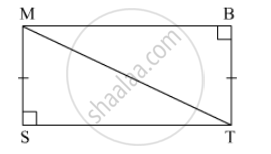SSC (English Medium) Class 8Maharashtra State Board
Share

# N Each Pair of Triangles Given Below, Parts Shown bOne Correspondence of Vertices by Which Triangles in Each Pair Are Congruent and Remaining Congruent Parts. - SSC (English Medium) Class 8 - Mathematics

ConceptCongruence of Triangles

#### Question

In the  triangle given below, parts shown by identical marks are congruent. State the test and the one to one correspondence of vertices by which triangles in each pair are congruent and remaining congruent parts.#### SolutionIn ∆MST and ∆TBM,
side MS ≅ side TB (given)
∠MST ≅ ∠TBM (right angle)
side MT is common
∴ By Hypotenuse-side test, ∆MST ≅ ∆TBM.
∴ side ST ≅ side MB,
∠SMT ≅ ∠BTM,
∠STM ≅ ∠BMT.

Is there an error in this question or solution?

#### APPEARS IN

Balbharati Solution for Balbharati Class 8 Mathematics (2019 to Current)
Chapter 13: Congruence of triangles
Practice Set 13.2 | Q: 1.1 | Page no. 87
Solution N Each Pair of Triangles Given Below, Parts Shown bOne Correspondence of Vertices by Which Triangles in Each Pair Are Congruent and Remaining Congruent Parts. Concept: Congruence of Triangles.
S